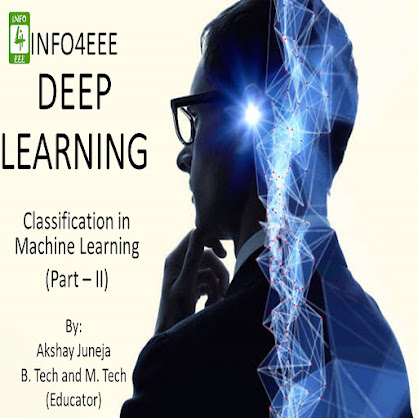Welcome back readers!! Last time we discussed four classification techniques. Moving on, other classification techniques are as follows:

#### Naïve Bayes

Hope you people remember Bayes Theorem to calculate probability from your schooling days (refer class 12th Mathematics Textbook). This classification is based on the same principle. The probability of a data element belonging to a particular class is independent of the presence of other data elements. Key points are as follows:

• Used for small datasets with multiple parameters
• Easy to interpret
• Used for better validation accuracy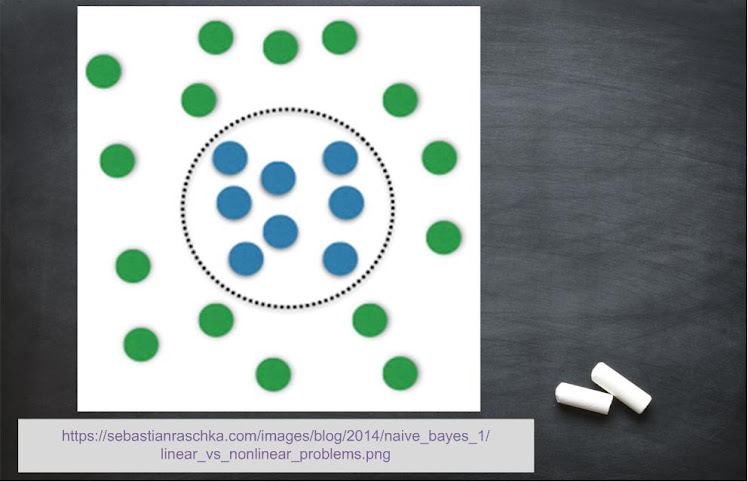#### Discriminant Analysis

This classification technique is based on Gaussian distribution or Normal distribution. The classification of data is performed by creating boundaries for each class and these boundaries are calculated using linear or quadratic function. Key points are as follows:

• Easy to interpret
• Less Memory
• Fast Prediction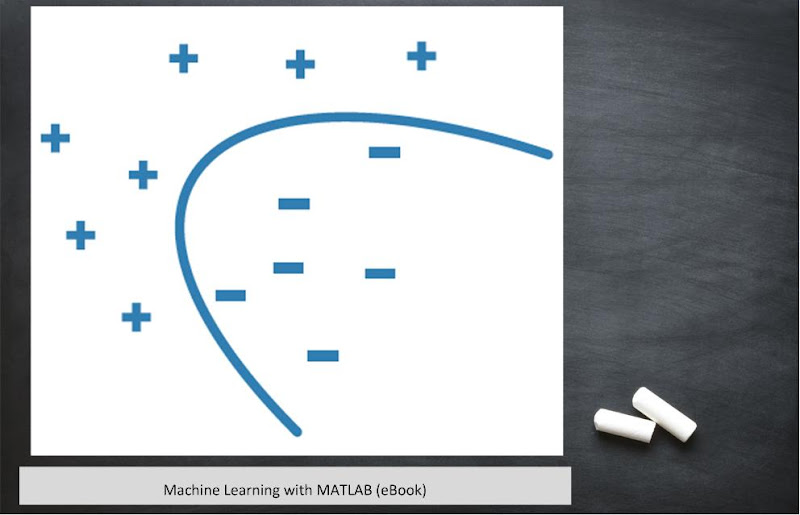#### Decision Tree

The data is classified on the basis of predicted responses. The structural representation is in form of a tree (not exactly like you see in a park), from a root node to a leaf node. The information of weights updating and number of branches is obtained while training the model. Detailed information about other nodes and modifications will be discussed in future articles. Key points are as follows:

• Fast and easy to interpret
• Less memory requirement
• High accuracy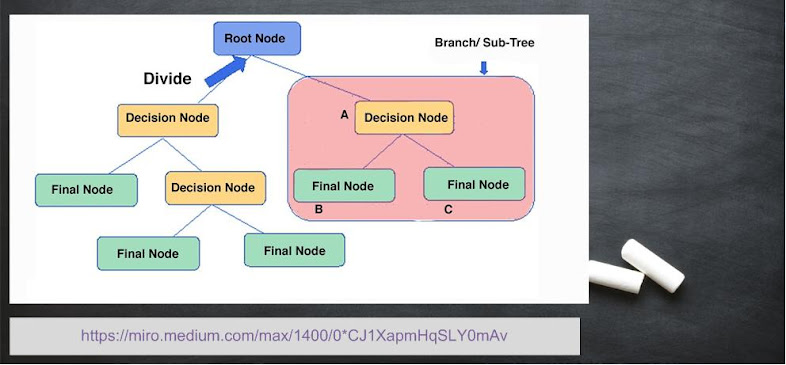#### Bagging and Boosting

They are categorized as ensemble machine learning algorithms. In these algorithms, the original dataset is divided into ‘N’ weak models. When bagging is implemented, ‘N’ sub-datasets are used to train ‘N’ weak models, one sub-dataset for each model. The class which is produced as output by maximum number of weak models is selected as final outcome. When boosting is implemented, complete dataset is used to train weak models one at a time and weights are updated after training each weak model. Key points are as follows:

• Used for systems with non-linear data
• Multiple models leads to more computation time is taken for training
• Bagging is used to reduce the complexity of overfitted models.
• As derived from the name, boosting is used to boost the performance of underfitted models.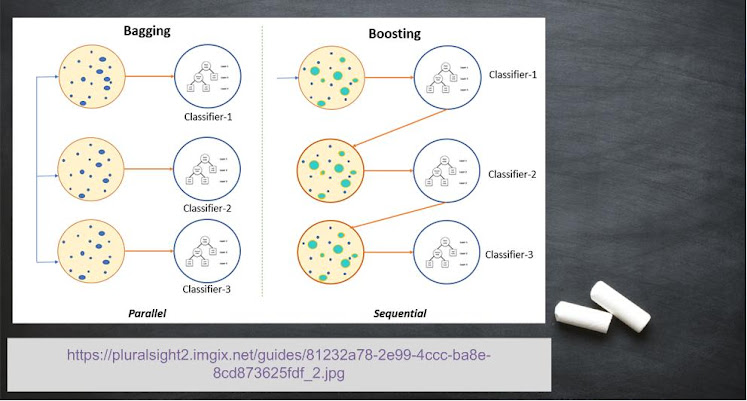So what we have is more than one decision tree. And what we call a place with more than one tree? A forest. In technical terms, a machine learning algorithm with more than one decision trees such that random features are extracted from each dataset, unlike bagging in which each decision tree is trained with complete dataset, is called a random forest.

So guys, this is all about classification techniques. From next article, we will start with regression-based machine learning algorithms.

But wait... Is it really over? Isn't it too less? Is this much information enough to understand classification-based techniques.

We are left with so many questions. Like What is weight? What are the various nodes in a tree? How a "machine" is learning all these things?

Well well well!!

This question will be answered in one of the future articles. Because I love to create suspense and unbox the mysteries at right time. I mean, who will watch all the seasons of a web series if the suspense is revealed in first few episodes of season 1 😄.